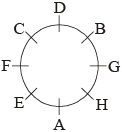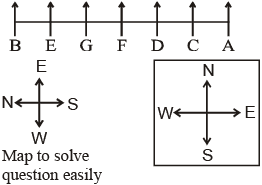### Seating Arrangements Tricks, Examples

In such problems a group of people, objects, etc, may have to be is arranged in a row or in a circle or any other way. Let us see the example given below:

Example: Just read the following informations carefully to answer the questions given below it:

 Five friends P, Q, R, S, and T are sitting on a bench:(i) P is sitting next to Q.(ii) R is sitting next to S.(iii) S is not sitting with T.(iv) T is on the last end of the bench.(v) R is on the 2nd position from the right.(vi) P is on the right of Q and T.(vii) P and R are sitting together.

1. Where is P sitting?

1. Between S and R
2. Between S and R
3. Between T and S
4. Between S and T
5. Between Q and R

2. Who is sitting in the centre?

1. P
2. Q
3. R
4. S
5. T

3. R is sitting between……………

1. Q and S
2. P and T
3. S and T
4. P and S
5. P and Q

4. What is the position of S?

1. Extreme left
2. Extreme right
3. Third from left
4. Second from left
5. None of these

5. What is the position of Q?

1. 2nd from right
2. Centre
3. Extreme left
4. 2nd from left
5. None of these

Now, point to be noted that in arrangement problems the actual information can be classified into 2 categories:

1. Definite information: A definite information is one when the place of object/man is definitely mentioned.
2. Comparative information: In such information the place of object/man is not mentioned definitely but only a comparative position is given. In other words the positions of objects/men are given in comparison to another objects/men.

Now, to solve the problem go as per the following steps:

• Step I: Sketch a diagram of empty places
• Step II: Fill up as many empty places as possible using all the definite informations.
• Step III: With the help of comparative information consider all possibilities and select the possibilities which does not violate any condition.

Now, we can solve the given example:

Here 4th and 5th sentences constitute definite information:

Comparative informations are: 1st, 2nd, 6th and 7th sentences while 3rd is a negative information.

T __ __ R __

Now, this is the time to look for the comparative informations that tell about T and R. Such informations are 2nd, 6th and 7th sentences. Take the 7th and the 1st sentence. If P and R are together and also Q and P are together, then P must be between Q and R. Now the arrangement take the form as:

T Q P R ____

By the virtue of the 2nd sentence:

T Q P R S

Now, look at the given questions and check that you get the following answer:

 1. (5) 2. (1) 3. (4) 4. (2) 5. (4)

### Solved Examples

Directions: Study the following information carefully and answer the question given below:

 A, B, C, D, E, F, G and H are sitting around a circle facing at the centre. D is second to the left of F and third to the right of H. A is second to the right of F and an immediate neighbour of H. C is second to the right of B and F is third to the right of B. G is not an immediate neighbour of F.

Question 1. How many of them, are there between H and C?

1. Two
2. Three
3. Two or Three
5. None of these

Question 2. Who is to the immediate left of A?

1. H
2. E
3. G
5. None of these

Question 3. In which of the following pairs is the first person sitting to the immediate left of the second person?

1. CD
2. BG
3. HA
4. FC
5. None of these

Question 4. Who is fourth to the right of B ?

1. E
2. C
3. A
5. None of these

Question 5. What is E’s position with respect to G?

1. Second to the right
2. Third to the left
3. Third to the right
4. Second to the left
5. None of these

Solution (Q. 1-5): Seating arrangement is as follows:Answers 1. (2) 2. (2) 3. (4) 4. (1) 5. (2)

Directions: On the basis of the information given below, select the correct alternative as answer for the questions which follow the information.

 (i) A, B, C, D, E, F and G are sitting on a wall facing east.(ii) C is just right of D.(iii) B is on end point and E is his neighbour.(iv) G is sitting between E and F.(v) D is third from south end.

Question 6.Which of the following is a pair of parsons who is on end points?

1. AE
2. AB
3. FB
4. CB

Question 7. Which of the following information is not necessary to determine the position of A?

1. i
2. ii
3. iii
4. All informations are necessary

Question 8. D is sitting between which of the following pairs?

1. CE
2. AC
3. CF
4. AF

Question 9. C want his seat as third from north, he will have to exchange the seat from which person?

1. G
2. F
3. E
4. Cannot be determined

Question 10. Who is seated right side of E ?

1. F
2. D
3. C
4. G

Solutions (6-10): Given information diagrammatically can be shown as follows:6. (2): A and B is a pair of persons who is at the end points.

7. (4): All informations are necessary

8. (3): D is sitted between C and F.

9. (4): He will have to exchange his seat from G.

10. (5): G is seated right side of E.